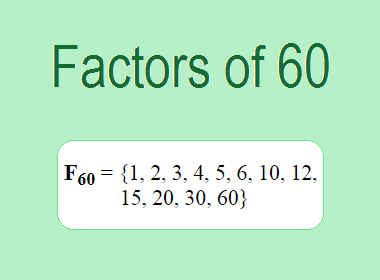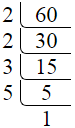# Factors of 60The factors of 60 are 1, 2, 3, 4, 5, 6, 10, 12, 15, 20, 30, and 60 i.e. F60 = {1, 2, 3, 4, 5, 6, 10, 12, 15, 20, 30, 60}. The factors of 60 are all the numbers that can divide 60 without leaving a remainder.

We can check if these numbers are factors of 60 by dividing 60 by each of them. If the result is a whole number, then the number is a factor of 60. Let's do this for each of the numbers listed above:

·        1 is a factor of 60 because 60 divided by 1 is 60.

·        2 is a factor of 60 because 60 divided by 2 is 30.

·        3 is a factor of 60 because 60 divided by 3 is 20.

·        4 is a factor of 60 because 60 divided by 4 is 15.

·        5 is a factor of 60 because 60 divided by 5 is 12.

·        6 is a factor of 60 because 60 divided by 6 is 10.

·        10 is a factor of 60 because 60 divided by 10 is 6.

·        12 is a factor of 60 because 60 divided by 12 is 5.

·        15 is a factor of 60 because 60 divided by 15 is 4.

·        20 is a factor of 60 because 60 divided by 20 is 3.

·        30 is a factor of 60 because 60 divided by 30 is 2.

·        60 is a factor of 60 because 60 divided by 60 is 1.

## How to Find Factors of 60?

1 and the number itself are the factors of every number. So, 1 and 60 are two factors of 60. To find the other factors of 60, we can start by dividing 60 by the numbers between 1 and 60. If we divide 60 by 2, we get a remainder of 0. Therefore, 2 is a factor of 60. If we divide 60 by 3, we get a remainder of 0. Therefore, 3 is also a factor of 60.

Next, we can check if 4 is a factor of 60. If we divide 60 by 4, we get a remainder of 0. Therefore, 4 is also a factor of 60. We can continue this process for all the possible factors of 60.

Through this process, we can find that the factors of 60 are 1, 2, 3, 4, 5, 6, 10, 12, 15, 20, 30, and 60. These are the only numbers that can divide 60 without leaving a remainder.

********************

********************

## Properties of the Factors of 60

The factors of 60 have some interesting properties. One of the properties is that the sum of the factors of 60 is equal to 168. We can see this by adding all the factors of 60 together:

1 + 2 + 3 + 4 + 5 + 6 + 10 + 12 + 15 + 20 + 30 + 60 = 168

Another property of the factors of 60 is that the prime factors of 60 are 2, 3, and 5 only.

## Applications of the Factors of 60

The factors of 60 have several applications in mathematics. One of the applications is in finding the highest common factor (HCF) of two or more numbers. The HCF is the largest factor that two or more numbers have in common. For example, to find the HCF of 60 and 24, we need to find the factors of both numbers and identify the largest factor they have in common. The factors of 60 are 1, 2, 3, 4, 5, 6, 10, 12, 15, 20, 30, and 60. The factors of 24 are 1, 2, 3, 4, 6, 8, 12, and 24. The largest factor that they have in common is 12. Therefore, the HCF of 60 and 24 is 12.

Another application of the factors of 60 is in prime factorization. Prime factorization is the process of expressing a number as the product of its prime factors. The prime factors of 60 are 2, 3, and 5 since these are the only prime numbers that can divide 60 without leaving a remainder. Therefore, we can express 60 as:

60 = 2 × 2 × 3 × 5

We can do prime factorization by division and factor tree method also. Here is the prime factorization of 60 by division method,60 = 2 × 2 × 3 × 5

Here is the prime factorization of 60 by the factor tree method,

Image 2

60 = 2 × 2 × 3 × 5

## Conclusion

The factors of 60 are the numbers that can divide 60 without leaving a remainder. The factors of 60 are 1, 2, 3, 4, 5, 6, 10, 12, 15, 20, 30, and 60. The factors of 60 have some interesting properties, such as having a sum of 168. The factors of 60 have several applications in mathematics, such as finding the highest common factor and prime factorization.